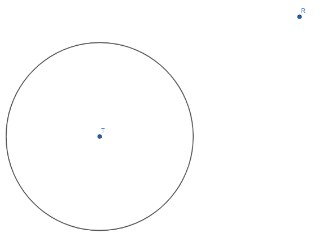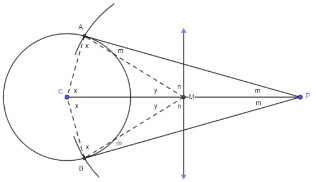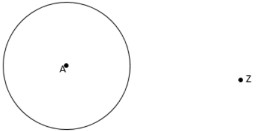# MA.912.GR.5.5Export Print
Given a point outside a circle, construct a line tangent to the circle that passes through the given point.

### Clarifications

Clarification 1: When given a circle, the center must be provided.

Clarification 2: Instruction includes using compass and straightedge, string, reflective devices, paper folding or dynamic geometric software.

General Information
Subject Area: Mathematics (B.E.S.T.)
Strand: Geometric Reasoning
Status: State Board Approved

## Benchmark Instructional Guide

• Circle

### Vertical Alignment

Previous Benchmarks

Next Benchmarks

### Purpose and Instructional Strategies

In grade 7, students learned about the circumference and area of a circle. In Geometry, students learn about tangents to circles, including using a compass and straightedge to construct a tangent that passes through a given point outside of the circle.
• Instruction includes the use of manipulatives, tools and geometric software. Allowing students to explore constructions with dynamic software reinforces why the constructions work.
• Instruction includes the student understanding that in a geometric construction, one does not use the markings on a ruler or on a protractor to construct inscribed and circumscribed circles of a triangle. Students should realize that there are limitations on precision that are inherent in the markings on rulers or protractors.
• It is important to build the understanding that formal constructions are valid when the lengths of segments or measures of angles are not known, or have values that do not appear on a ruler or protractor, including irrational values.
• Instruction includes the connection to logical reasoning and visual proofs when verifying that a construction works.
• Instruction includes the connection to inscribed angles in circles; to the lengths of tangents drawn from an external point to a circle; to circumcenters of right triangles; and to a tangent of a circle (understanding that it is perpendicular to the radius at the point of tangency). (MTR.5.1)
• To construct line tangent to a circle that passes through the given point, students can start by drawing a segment from the center, C, to an external point, P. Students can then determine the midpoint, M, of CP by constructing the perpendicular bisector. Then, students can set the compass width equal to the distance from point C to point M and place the compass point at point M to create circle M. Students should realize that circle M intersects circle C at two points, A and B. Using properties of circles and inscribed angles, students should be able to conclude that angle PAC and angle PBC are right angles. Since the line containing points P and A is perpendicular to a radius, AC, of circle C, it is a tangent line passing through point P. Similarly, the line containing points P and B is also a tangent line.
• For expectations of this benchmark, constructions should be reasonably accurate and the emphasis is to make connections between the construction steps and the definitions, properties and theorems supporting them.
• While going over the steps of geometric constructions, ensure that students develop vocabulary to describe the steps precisely. (MTR.4.1)
• Problem types include identifying the next step of a construction, a missing step in a construction or the order of the steps in a construction.

### Common Misconceptions or Errors

• Students may try to construct the tangent line by placing a straightedge from the external point to a point where it just touches the circle. To help address this, discuss with students how they could justify whether it will only touch the circle at one point without knowing which point that is.

• Circle T and an external point R are provided below.• Part A. Fill in the blank to complete the sentence below. If a line is tangent to a circle it is ____________ to the radius of the circle at the point of tangency.
• Part B. Draw segment RT.
• Part C. Bisect segment RT and label the midpoint S.
• Part D. Construct circle S using ST as the length of the radius. In how many places does circle S intersect circle T?
• Part E. Label one of the points of intersection, as described in Part D, V. What is the measure of angle TVR?
• Part F. What is the relationship between TV and line RV?
• Part H. What can you conclude about line PV and how it relates to circle T?

• A picture is given below that shows how Delia constructed a line tangent through point P to circle C.• Part A. If circle C and point P were originally given, describe how Delia could have constructed points M, B and A.
• Part B. Classify triangle AMC, triangle BMC, triangle AMP and triangle BMP.
• Part C. Delia labeled certain angle measures as $x$, $m$, $y$ and $n$ indicating that certain pairs of angles are congruent. Justify her placement of these angle measures and their congruency.
• Part D. Using the Triangle Sum Theorem two equations relating the angle measures for triangle AMC and the angle measures for triangle AMP.
• Part E. Use your equations from Part D to show that 2$x$ + 2$m$ + $y$ + $n$ = 360°.
• Part F. What do you notice, and can you conclude, about $y$ and $n$?
• Part G. Using the equation from Part E and your conclusion from Part F, show that $x$ and $m$ are complementary angles.
• Part H. How does your result from Part G show that Delia correctly constructed the tangent line, AP, to circle C?

### Instructional Items

Instructional Item 1
• Construct a line tangent to circle A that goes through point Z.*The strategies, tasks and items included in the B1G-M are examples and should not be considered comprehensive.

## Related Courses

This benchmark is part of these courses.
1200400: Foundational Skills in Mathematics 9-12 (Specifically in versions: 2014 - 2015, 2015 - 2022, 2022 and beyond (current))
1206320: Geometry Honors (Specifically in versions: 2014 - 2015, 2015 - 2022, 2022 and beyond (current))

## Related Access Points

Alternate version of this benchmark for students with significant cognitive disabilities.

## Related Resources

Vetted resources educators can use to teach the concepts and skills in this benchmark.

## Formative Assessments

Using a Compass To Construct a Tangent Line:

Students are asked to construct a tangent line to a given circle from a given exterior point.

Type: Formative Assessment

Constructing a Tangent Line:

Students are asked to complete and justify the construction of a line tangent to a circle from an exterior point.

Type: Formative Assessment

## MFAS Formative Assessments

Constructing a Tangent Line:

Students are asked to complete and justify the construction of a line tangent to a circle from an exterior point.

Using a Compass To Construct a Tangent Line:

Students are asked to construct a tangent line to a given circle from a given exterior point.

## Student Resources

Vetted resources students can use to learn the concepts and skills in this benchmark.

## Parent Resources

Vetted resources caregivers can use to help students learn the concepts and skills in this benchmark.